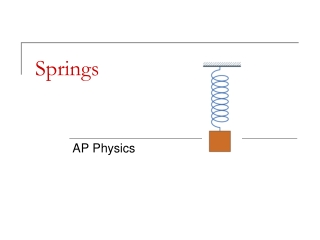DownloadDownload PresentationSprings

# Springs

Télécharger la présentation## Springs

- - - - - - - - - - - - - - - - - - - - - - - - - - - E N D - - - - - - - - - - - - - - - - - - - - - - - - - - -
##### Presentation Transcript

1. Springs AP Physics

2. Force on a Spring • When you apply a force to a spring or a rubber band, it stretches. • A scientist would ask, "How is the force that you apply related to the amount of stretch?" • This question was answered by Robert Hooke, a contemporary of Newton, and the answer has come to be called Hooke's Law.

3. Simple Harmonic Motion – Repetitive Motion • Back and forth motion that is caused by a force that is directly proportional to the displacement. The displacement centers around an equilibrium position.

4. Springs – Hooke’s Law • One of the simplest type of simple harmonic motion is an oscillating SPRING.

5. Restoring Force – Why negative? • The negative sign indicates that the spring force is a restoring force. • Fs always acts in the opposite direction from the direction in which the system is displaced. • Fs is force necessary to get back to equilibrium. • you may have to make the force negative. • The origin has to be placed at the position where the spring system would be in static equilibrium. • This is the location were the net force on the object to which the spring is attached is equal to zero.

6. Stretch/Compression and the Spring • Stretch – pulling of spring to elongate • Positive displacement (+x) • Compression – pushing on spring to shorten • Negative displacement (-x) • The spring constant (k) represents the stiffness of the spring. • Larger the constant  harder to stretch

7. This is the formula you will see and use for Hooke’s Law: Common formulas which are set equal to Hooke's law are: Hooke’s Law

8. What does the slope tell you? Hooke’s Law from a Graphical Point of View Suppose we had the following data:

9. Example • A weight of 50 N is attached to a spring hanging vertically stretches the spring 5.0 cm. The spring is now placed horizontally on a table and stretched 11.0 cm. What force is required to stretch the spring this amount? -110 N 1000 N/m

10. We have seen F vs. x Before!!!! Work or ENERGY = FDx Since WORK or ENERGY is the AREA, we must get some type of energy when we compress or elongate the spring. This energy is the AREA under the line! Area = ELASTIC POTENTIAL ENERGY Since we STORE energy when the spring is compressed and elongated it classifies itself as a “type” of POTENTIAL ENERGY, Us. In this case, it is called ELASTIC POTENTIAL ENERGY.

11. Elastic Potential Energy • The graph of F vs.x for a spring that is IDEAL in nature will always produce a line with a positive linear slope. Thus the area under the line will always be represented as a triangle. NOTE: Keep in mind that this can be applied to WORK or can be conserved with any other type of energy.

12. Conservation of Energy in Springs

13. Horizontal Spring Problem • A 10 kg box is pushed against a horizontal spring by a force of 100 N compressing the spring 10 cm. • Ignoring friction, what is the speed of the box through equilibrium? • With a coefficient of friction of 0.2 between the box and the horizontal surface, what is the speed of the box through equilibrium? 1.0 m/s 0.78 m/s

14. Vertical Spring Problem • A 250 g block is placed on a vertical spring (k = 5000 N/m) and pushed downward, compressing the spring 10 cm. The block is released, the spring launched the block into the air. • What is the speed of the block as it leaves the spring? • What height above the point of release from spring will the block reach? 14.1 m/s 10.1 m

15. Graph the Energy for the following: • An object is falling through the air • A mass oscillating up and down on a spring (ignoring air resistance) • A crate is accelerated from rest across a horizontal, rough surface • A block is pushed and then released across a horizontal, rough surface Potential? Kinetic? Heat?

16. Ramps • How do you account for the energy changes of the block-spring system on a ramp?

17. Path Dependence

18. The Oscillating Spring • The FREQUENCY of a wave is the inverse of the PERIOD. That means that the frequency is the #cycles per sec. The commonly used unit is HERTZ (Hz). • Hz = 1/s